# String Matching and Database Merging

Machine Learning to compare and join heterogeneous data from heterogeneous sources.

Merging databases is a complicated business, names are sometimes different, even when they refer to the same thing (see examples below). This is caused by spelling mistakes, different conventions, etc.

Here I merge two databases of movie/shows records to compare different string matching methods. I find that machine learning performs better than any single algorithm by itself, although some of them are pretty close. The data itself is very noise and therefore difficult to characterize. Some examples:

B-Side vs. B-Sides: Match

Baa Baa Black Sheep, Vol. 1 vs. Baa Baa Black Sheep: Match

G-Force (Blu-ray 3D/ DVD & Blu-ray Combo) vs. G Force: Match

D. Gray-Man: Season 1 vs. D.Gray-man: Match

C’Mon Man vs. Con Man: Mismatch

E-40 vs. E:60: Mismatch

# Introduction

All the methods are based on comparing strings. Figure 1 shows a nice overview by Felix Naumann (great powerpoint if you’re interested in the math). I’ll explain without math the ones I used.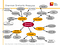Figure 1. Overview similarity measures

Edit-based measures: Based on characters. The distance is proportional to the effort it takes to convert one string into the other.

• Levenshtein distance: Distance proportional number of single-character edits (i.e. insertions, deletions or substitutions) to convert one string into the other.
• Jaro distance: Distance dependent on the number of matching characters and transpositions.
• Jaro-Winkler distance: Similar, but different parts of the string weight different, resulting on a better performance for short strings.
• Sørensen index or dice’s coefficient: Quotient between the number of characters shared and the lengths of the two strings.
• Jaccard index: Equivalent to the dice’s coefficient. J = D/(2-D).

Token-based measures: Each string is divided into words or n-grams (substrings of consecutive letters). Two strings are similar if they have exact words or n-grams in common

• Dice’s coefficient: Quotient between the number of n-grams shared and the total number of n-grams in the two strings.
• Cosine similarity: Each string is represented as a vector, where the dimensions correspond to the different possible words/n-grams. The dimensions are usually weighted with TF-IDF (term frequency–inverse document frequency). Words/n-grams that appear many times in a string, but only a few times in the set of strings are weight more. The cosine of the angle between the two vectors is the distance measure.

Hybrid: Similar to a token-based measures, but words/n-grams in two different words are considered a partial match if they are similar (using an edit-based measure for example). SOFT TF-IDF fits here.

Phonetic: Each string is converted to a phonetic representation. Good with names, since several names sound similar and can be mistaken. Language dependent.

Domain-dependent: Using other attributes. For example the year of the movie. Since I try to generalize a bit I won’t use these.

Wikipedia “Machine learning explores the construction and study of algorithms that can learn from and make predictions on data.”

I used machine learning to combine the distance measures above.

There are two main classes:

• Unsupervised: The algorithm learns and predict at the same time. For example in clustering you give some points to the algorithm and a number of clusters and it divides the points in the best possible way.
• Supervised: You need to train the algorithm to use it later. For example in classification you need to give them some examples already classified for the algorithm to be able to predict new values.

I will focus on the later, since I’m trying to predict if two strings are the same or not. I used the Python library sklearn for this. To use and examine the algorithms I need training and testing datasets. For this, I matched pairs of movies in the two database using the Jaro distance, and then manually labeled around 800 if the match was correct or incorrect (probably introducing some mistakes in the way). I splitted the data in 300 for the training and 500 for the testing.

• Support vector machine (SVM): Geometry based, tries to find a group of “planes” that divides our set of points accordingly to its class, in a way that the space between the points and the “planes” is maximum. The “planes” can be linear or not, depending on the kernel used (Figure 2). I used a linear kernel.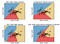Fgure 2. Different kernels in Supoort vector classification. Source: sklearn
• Logistic regression: Probabilistic model, it assumes a “S” shaped curve. Behaves similarly than LinearSVC in practice.

Both models minimize a cost function, with two terms (1) Loss = How well the train data is classified (2) Penalty = How complicated the model is. Since I have many redundant measures of string similarity, I used an L1-norm for the penalty, which often learn to ignore them and place more weight on the more important coefficients.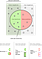Figure 3. Quality measures

Finally, we need a way to measure quality of prediction. There are many measures (Fig. 3), but two of the most important are F1-score and ROC-curve.

Precision: Among the ones the algorithm says are positive, what percentage are true positive?

True positive rate (or recall): Among all the positive, what percentage are labeled as positive by the algorithm?

False positive rate: Among all the negative, what percentage are labeled as positive by the algorithm?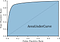Figure 4. ROC curve

Area under the curve of the receiver operating characteristic (ROC curve, fig. 4). The ROC curve plots the false positive rate versus the true positive rate (recall).

F1-score: The harmonic mean of precision and recall.

# Results:

Figure 5 shows the AUC and F1-scores for all the methods used against the testing data. The testing data contains pairs of movies, and around 20% of the pairs correspond to the same movie.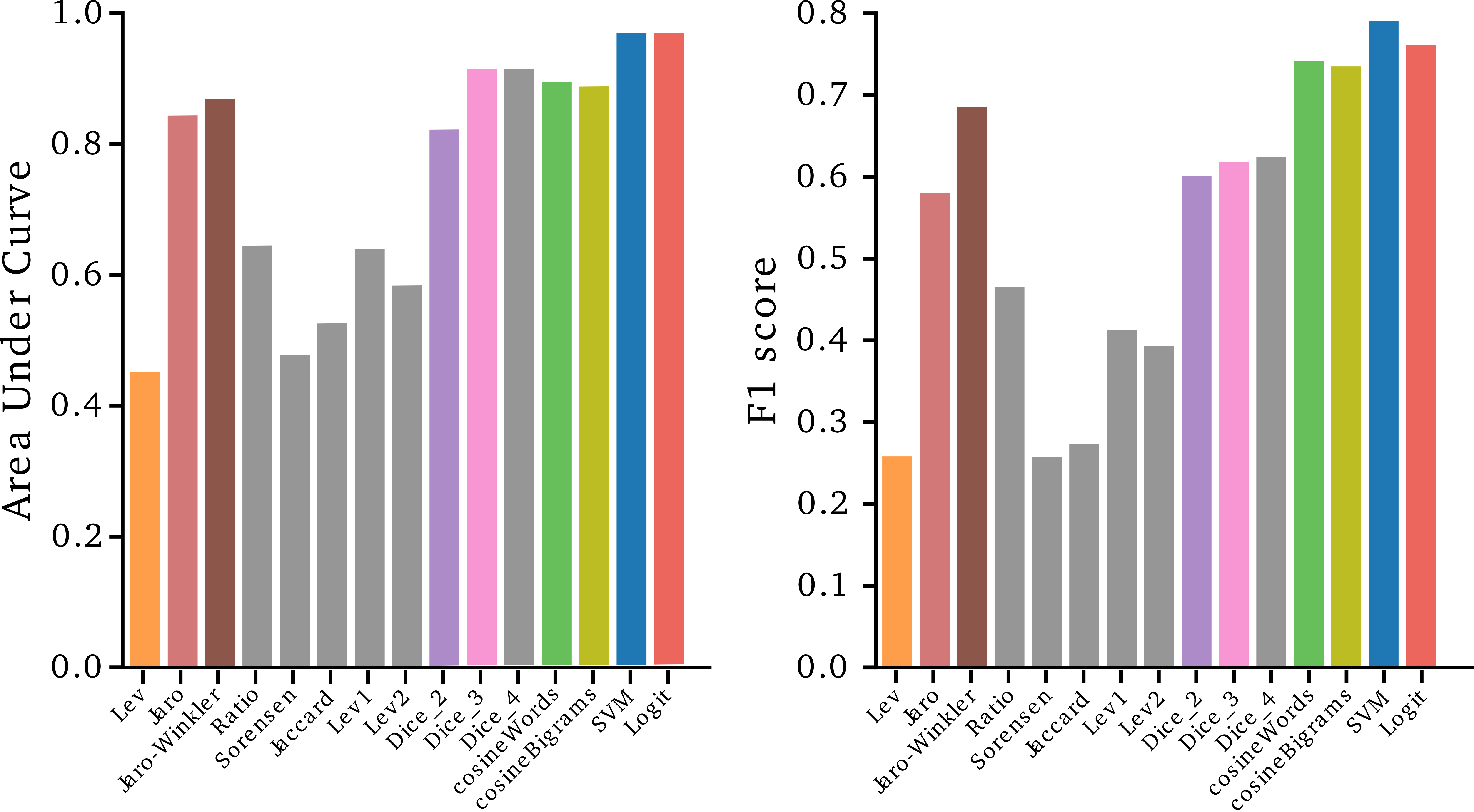Figure 5. AUC and F1 for all methods

We can see (Figs. 5, 6):

1. Edit-based measures are generally bad
• Makes sense. “Star Wars” and “Star Wars: Return of the Jedi” are very different if we attend only to characters.
• The exception is Jaro-Winkler, which is really good. This is because the extra “Return of the Jedi” doesn’t have a lot of weight in this algorithm.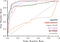Figure 6. ROC curves

2. Token-based measures are usually good

• Using words works as well as N-grams.
• Cosine similarity (TF-IDF) works a little better, especially if having a low false positive rate is very important for you (Fig. 6).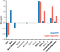Figure 7. Weights of the features in the Machine Learning models

3. Machine learning works the best

• Expected, since they combine both measures.
• Interestingly, they use some measures that are not the best by themselves. For example the Levenshtein distance, which is horrible by itself is needed for a good prediction (Fig. 7).

This is an example for one database. Using other measures may be better, and the overhead of using machine learning may not be worth it in some situations.

Data Juggler. Computational Scientist. www.javiergb.com

## More from Javier GB

Data Juggler. Computational Scientist. www.javiergb.com